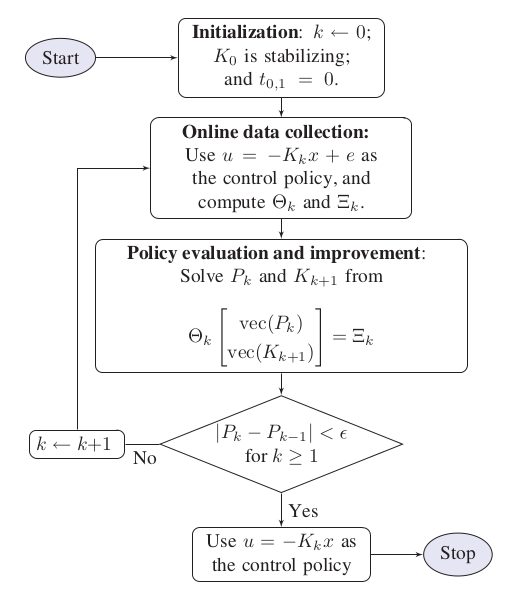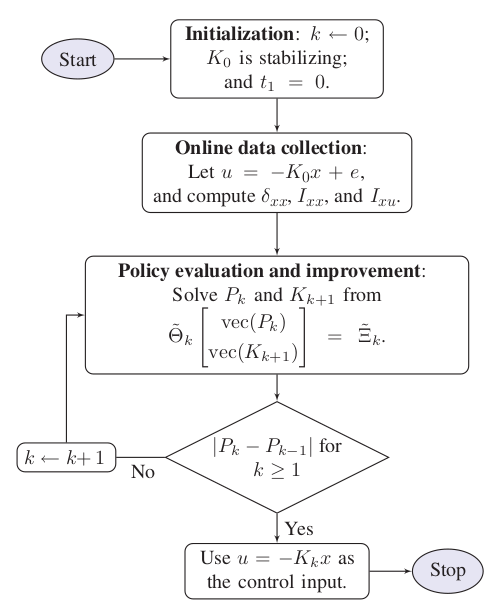The below algorithms is given in the book Robust Adaptive Dynamic Programming.

## Problem statements¶

Given the linear system equation (1), design a linear quadratic regulator (LQR) in the form ofthat minimizes the following cost functionwhere.

The solution of this problem iswhich is also the solution of the Riccati equation, can be obtained by OpenControl.ADP_control.LTIController.LQR(). However, this approach requires the knowledge of the system dynamic (the matrix A and B). The model-free approach below will resolve this problem.

Lets begin with another control policywhere the time-varying signal e denotes an artificial noise, known as the exploration noise. Taking time derivative of the cost function and integrate it within intervalto obtain:

(1)where.
We can see that this is the fix point equation, meaning that the K matrix can be solve by finite number of iteration. And because of no requirement of the system dynamic, it is a data-driven approach.

## On-policy learning¶

For computational simplicity, we rewrite (1) in the following matrix form:

(2)whereNote

• To adopt the persistent excitation condition, one must choose a sufficiently large number of datato satisfy the following condition:### Algorithm¶### Library Usage¶

Setup a simulation section with OpenControl.ADP_control.LTIController and OpenControl.ADP_control.LTIController.setPolicyParam() then perform simulation by OpenControl.ADP_control.LTIController.onPolicy()

from OpenControl.ADP_control import LTIController

Ctrl = LTIController(sys)
# set parameters for policy
Q = np.eye(3); R = np.array([]); K0 = np.zeros((1,3))
explore_noise=lambda t: 2*np.sin(10*t)
data_eval = 0.1; num_data = 10

Ctrl.setPolicyParam(K0=K0, Q=Q, R=R, data_eval=data_eval, num_data=num_data, explore_noise=explore_noise)
# take simulation and get the results
K, P = Ctrl.onPolicy()


## Off-policy learning¶

Let define some new matricesanddefined by:then for any given stabilizing gain matrix, (1) implies the same matrix form as (2)

### Algorithm¶### Library Usage¶

Setup a simulation section the same as the section then perform simulation by OpenControl.ADP_control.LTIController.offPolicy()

K, P = Ctrl.offPolicy()